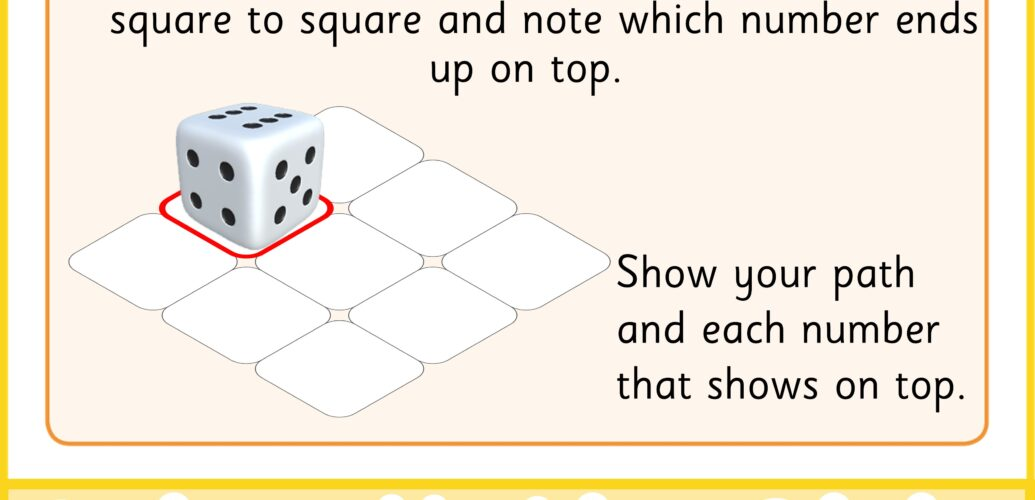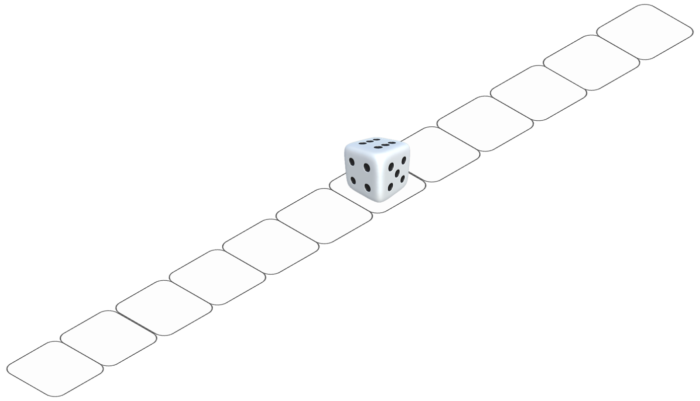# Dice and Spatial Reasoning

We generally think of dice as a way of generating a random number but dice are actually interesting shapes, even more so than just the cube (or other solid) that they are created from. This week we are exploring how the arrangement of numbers and the shapes involved in dice can be explored. If you would like to try these challenges in our online maths clubs, visit https://online.roots2grow.org/ and book a free trial session.

What action is associated with dice? We can throw them, which is sensible enough for any object the size of an average die, or we can roll them which is a little strange for an object that is generally a cube. But roll them we do. And rotation is the motion that occurs.

Can we imagine that die rotating? Can we predict which numbers will appear? Are there any patterns that will help us understand how the numbers are arranged? Perhaps we could imagine it as a stamp that stamps whichever number is facing down or touching the paper.What numbers would get printed on this track by the dice if it were a stamp?

Some related topics:

• Transformations (rotation)
• Number bonds (to 7, 13 etc.)
• Formulae (working out edges and vertices)
• Geometry (platonic solids)
• Polyhedra and nets

To try this activity: